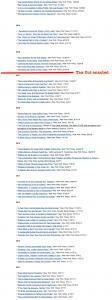# Archive | visuals

## taxes and incentives at work

For some reason I remembered yesterday that opinion by Greg Mankiwfrom 2010 where he warned us that the (then feared) tax hikes by Obama admin would diminish his personal incentives to work. He was talking very directly about his output as articles which we would miss: to quote, “I [would] supply less of my services[…;] because you are reading this article, you are one of my customers.”

I thought it would be interesting to check what the 2017′ Tax Cuts and Jobs Act did to Mankiw’s productivity.…

## alons enfants de la patrie

In collaboration with Cynthia Sung, Daniel Feshbach and bloodthirsty French people……

## Mapping department strength

What is your math department strength? One of those rather polarizing questions, or an opportunity to gossip without guilt.

Still, important to understand where your department is on the map, if one wants to steer it in some particular direction, or to keep it where it is.

I wrote a short python script, using BeautifulSoup (thank you!) and campus-wide subscription to MathSciNet (thank you too!) – and downloaded the raw numbers: how many items (whichever MathSciNet is indexing: articles, books, theses,…) are authored by folks from ‘1-IL’ (our code) vs.…

## Shuffling the sheep

$$latex \def\xx{\mathbf{x}}\def\Real{\mathbb{R}}$$

We consider the problem of flock control: what are the natural constraints on the steering agents in reconfiguring an ensemble of agents?

Our basic setup is following: the agents are modeled as points $latex \xx=(x_1,\ldots,x_n), x_k\in X, k=1,\ldots, N$, with $latex X$ some terrain (for simplicity, we constrain ourselves here to Euclidean plane, but in general it can be some reasonably tame subset of a manifold). Quasi-static behavior is assumed, under which the system, for each value of the controlling parameters quickly settles into (locally minimizing) the equilibrium whose basin of attraction contains current position.…

## Protected: Curvilinear Origami

There is no excerpt because this is a protected post.

## Topologically constrained models of statistical physics.

$$\def\Real{\mathbb{R}} \def\Int{\mathbb{Z}} \def\Comp{\mathbb{C}} \def\Rat{\mathbb{Q}} \def\Field{\mathbb{F}} \def\Fun{\mathbf{Fun}} \def\e{\mathbf{e}} \def\f{\mathbf{f}} \def\bv{\mathbf{v}} \def\blob{\mathcal{B}}$$

### The Blob

Consider the following planar “spin model”: the state of the system is a function from $$\Int^2$$ into $$\{0,1\}$$ (on and off states). We interpret the site $$(i,j), i,j\in\Int$$ as the plaque, i.e. the (closed) square given by the inequalities $$s_{ij}:=i-1/2\leq x\leq i+1/2; j-1/2\leq y\leq j+1/2$$.

To any configuration $$\eta$$ we associate the corresponding active domain,
$A_\eta=\bigcup_{(i,j): \eta(i,j)=1} s_{ij}.$

We are interested in the statistical ensembles supported by the finite contractible active domains – let’s refer to such domains as blobs.…

## dimension of the Internet

As reported in the talk on the hyperbolic geometry of maps and networks, the often claimed approximability of the Internet graph (the routing graph as seen by Border Gateway Protocol) by samples from disks of large radius in the hyperbolic plane is somewhat questionable: the local dimensions of the Rips complexes build from the ASN graphs are wild.

Below are links to Javascript based visualizations
(designed and implemented by Yuriy Mileyko)
of the Rips complexes derived from